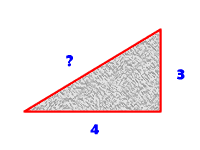# Use the Pythagorean Theorem for Right Triangles...

### Question:

If I'm given a right triangle and two of its sides, how can I find the length of the third side? Can I do this if it's not a right triangle?

Finding the missing side of a right triangle is a pretty simple matter if two sides are known. One of the more famous mathematical formulas is $$a^2+b^2=c^2$$, which is known as the Pythagorean Theorem. The theorem states that the hypotenuse of a right triangle can be easily calculated from the lengths of the sides. The hypotenuse is the longest side of a right triangle.If you're given the lengths of the two sides it is easy to find the hypotenuse. Just square the sides, add them, and then take the square root. Here's an example:Since we are given that the two legs of the triangle are 3 and 4, plug those into the Pythagorean equation and solve for the hypotenuse:

$$a^2+b^2=c^2$$ $$3^2+4^2=c^2$$ $$25 = c^2$$ $$c = \sqrt{25}$$ $$c = 5$$

If you are given the hypotenuse and one of the legs, it's going to be slightly more complicated, but only because you have to do some algebra first. Suppose you know that one leg is 5 and the hypotenuse (longest side) is 13. Plug those into the appropriate places in the Pythagorean equation:

$$a^2+b^2=c^2$$ $$5^2+b^2=13^2$$ $$25+b^2=169$$ $$b^2=144$$ $$b = 12$$

As you can see, it is pretty simple to use the Pythagorean Theorem to find the missing side length of a right triangle. But -- what if it's not a right triangle? If you change that angle in the triangle there can obviously be any number of possibilities for the hypotenuse! Thus, you need more information to solve the problem. You can try using the Law of Sines or the Law of Cosines to determine side lengths in other triangles.